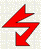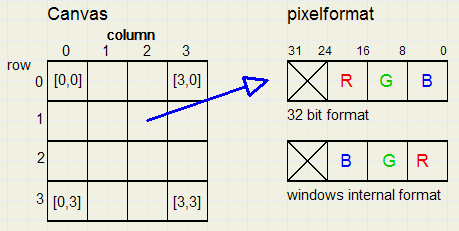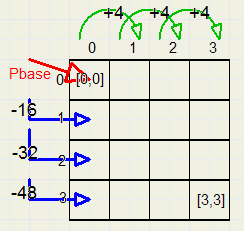Delphi drawing, part 1contents

 part1 modifying pixels in a bitmap part2 drawing dots and lines: the XBitmap class part3 flicker free painting part4 drawing circles and ellipses

Introduction

In this part - 1, the very basic principles are covered : single pixels.
The described methods may be used as the basis for more complicated geometry.
And, by having full control of the drawing process, the programmer may add enhancements
that Delphi does not offer, such as:
- dash-dot lines of greater width than 1 pixel
- lines that change color on the way
- canvasses with several levels
- use of a clipping rectangle
- slow motion drawing for educational purposes
Part-2, to be published later, will cover lines and ellipses, clipping rectangles, large penwidths dash-dot lines
and 4 level drawing planes.

In Delphi, several ways exist to draw lines or fill shapes.

Ultimately, all drawing amounts to setting a single pixel on a bitmap canvas.
Therefore, single pixels are written and the time required is measured.
Each drawing method is used in two type of actions
1. drawing a line
2. filling a rectangle with a color

On the Form, several BitButtons are placed.
Pressing a button triggers a specific action and the time needed per pixel is indicated
in the Statictext.

All drawing is done in a 100 * 100 bitmap, named bm.
The pixelformat is 32 bit.
A paintbox is added to show the contents of the bitmap after drawing.

The time is obtained from the system clock, which counts milliseconds,
by the GetTickCount function.
For accurate measurement, the operation has to be repeated many times.
Procedure ShowTime(n) presents the elapsed time after writing n pixels.

Bitmap Layout
Figure below shows the way pixels are organised in the bitmap.Pixels are represented by 32-bit unsigned words.
Pixels are accessable by their horizontal- and vertical indexes.
Note the difference between the way the picture is stored in the bitmap
and the internal windows format.
The statement
pixvalue := bm.canvas.pixels[2,3]
reads the pixel from column 2, row 3 , converts the format to windows internal
and stores this value in variable pixvalue, which must be of type LongInt or DWORD.

Another way to acces the pixels is by using a pointer to the first element of each row.
The ScanLine property of the bitmap provides this pointer.
Now, we can acces a selected row in the same way as an array.
```type TA = array[0..1000] of DWORD;
PA = ^TA;
var p : PA;
begin
p := bm.scanline; // p points to pixel [0,1]
p^ := \$ff00ff; // set [2,1] to purple color
.......
.......
end;
```
The ScanLine property provides a much faster access of the bitmap,
especially when many operations on the same row take place.
Note: by directly accessing the bitmap, the 32-bit format is used, no conversion
to the internal windows format takes place.

As we will see from the time measurements later, the scanline statement itself
is rather time consuming.
Therefore, more time can be saved by minimizing its use.
See figure below, showing pointers to the memory storing the bitmapWhen pointer Pbase points to the memory location of pixel [0,0],
Pbase + 4 points to [1,0] and Pbase - 16 points to [0,1]

Using ScanLine only once to obtain Pbase, we can access pixels in the following way
```//assume bitmap width of 100 pixels, 32 bit format
type PDW = ^DWORD;
var Pbase,Pline,p : PDW;
begin
Pbase := bm.scanline;
Pline := PDW(DWORD(Pbase) - y * 400);//Pline points to [0,y] as scanline did before
p := PDW(DWORD(Pline) + (x shl 2));//p points to element x on selected row
p^ := \$ff00ff;//set pixel [x,y] to color purple
.........
........
end;
```

Drawing Lines
The "pixels[ ]" method
 ```procedure TForm1.BitBtn1Click(Sender: TObject); //pixels line drawing //paint 1000000 pixels var i : longInt; k : byte; begin clearBm; t1 := gettickCount; with bm do with canvas do for i := 1 to 10000 do for k := 0 to 99 do pixels[k,k] := \$0; t2 := gettickcount; showtime(1000000); end;```
The "LinoTo" method
 ```procedure TForm1.BitBtn8Click(Sender: TObject); //lineto drawing var i : longInt; begin clearBm; t1 := gettickCount; with bm do with canvas do begin pen.Color := \$00ff00; for i := 1 to 1000000 do begin moveto(0,0); lineto(100,100); end; end; t2 := gettickcount; showtime(100000000); end;```
The "ScanLine" method
 ```procedure TForm1.BitBtn3Click(Sender: TObject); //scanline line drawing type PDW = ^DWORD; var i : integer; k : byte; p,pbase : PDW; begin clearBM; t1 := gettickCount; for i := 1 to 10000 do for k := 0 to 99 do begin pbase := bm.scanline[k]; p := PDW(DWORD(pbase) + (k shl 2)); p^ := \$ff00ff; end; t2 := gettickcount; showtime(1000000); end;```
The "Xdot" method
 ```procedure TForm1.BitBtn2Click(Sender: TObject); //xdot line drawing type PDW = ^DWORD; var i : integer; k : byte; p,pbase : PDW; begin clearBM; t1 := gettickCount; pbase := bm.scanline; for i := 1 to 1000000 do for k := 0 to 99 do begin p := PDW(DWORD(pbase) + (k shl 2)- k * 400); p^ := \$0080ff; end; t2 := gettickcount; showtime(100000000); end;```
Filling Rectangles
The "pixels[ ]" method
 ```procedure TForm1.BitBtn4Click(Sender: TObject); //fill bm pixel by pixel var x,y : byte; k : integer; begin t1 := gettickcount; with bm do with canvas do for k := 1 to 100 do for y := 0 to 99 do for x := 0 to 99 do pixels[x,y] := \$ff0000; t2 :=gettickcount; showtime(1000000); end;```
The "FillRect" method
 ```procedure TForm1.BitBtn5Click(Sender: TObject); //fillrect bm var n : integer; begin with bm do with canvas do begin brush.style := bssolid; brush.color := \$808080; t1 := gettickcount; for n := 0 to 9999 do fillrect(rect(0,0,width,height)); t2 := gettickcount; end; showtime(100000000); end;```
The "ScanLine" method
 ```procedure TForm1.BitBtn6Click(Sender: TObject); //scanline fill timing type PDW = ^DWORD; var i : integer; x,y : byte; k : word; p,pbase : PDW; begin clearBM; t1 := gettickCount; for k := 1 to 10000 do for y := 0 to 99 do begin pbase := bm.scanline[y]; for x := 0 to 99 do begin p := PDW(DWORD(pbase) + (x shl 2)); p^ := \$ff00ff; end; end; t2 := gettickcount; showtime(100000000); end;```
The "XfillRect" method
 ```procedure TForm1.BitBtn7Click(Sender: TObject); //xdot fill timing type PDW = ^DWORD; var x,y : byte; k : word; p,pbase,pline : PDW; begin clearBM; t1 := gettickCount; pbase := bm.scanline; for k := 1 to 10000 do for y := 0 to 99 do begin pline := PDW(DWORD(pbase) - y*400); for x := 0 to 99 do begin p := PDW(DWORD(pline) + (x shl 2)); p^ := \$0080ff; end; end; t2 := gettickcount; showtime(100000000); end;```
Time measurements
Times are in nanoseconds per pixel.
 . line fill pixels[ ] 720 720 lineto,fillrect 13,75 1,25 scanline 328 5,93 Xdot,Xfill 4,68 2,66
The remaining part of the test program
 ```unit Unit1; interface uses Windows, Messages, SysUtils, Classes, Graphics, Controls, Forms, Dialogs, StdCtrls, Buttons, ExtCtrls; type TForm1 = class(TForm) PaintBox1: TPaintBox; StaticText1: TStaticText; Label1: TLabel; BitBtn1: TBitBtn; BitBtn2: TBitBtn; BitBtn3: TBitBtn; Label2: TLabel; Label3: TLabel; BitBtn4: TBitBtn; BitBtn5: TBitBtn; BitBtn6: TBitBtn; BitBtn7: TBitBtn; BitBtn8: TBitBtn; procedure FormCreate(Sender: TObject); procedure FormDestroy(Sender: TObject); procedure PaintBox1Paint(Sender: TObject); procedure BitBtn1Click(Sender: TObject); procedure BitBtn2Click(Sender: TObject); procedure BitBtn3Click(Sender: TObject); procedure BitBtn4Click(Sender: TObject); procedure BitBtn5Click(Sender: TObject); procedure BitBtn6Click(Sender: TObject); procedure BitBtn7Click(Sender: TObject); procedure BitBtn8Click(Sender: TObject); private { Private declarations } public { Public declarations } end; var Form1: TForm1; implementation {\$R *.DFM} var bm : Tbitmap; t1,t2 : LongInt; procedure showtime(t : double); //t = number of pixels processed begin form1.paintbox1.invalidate; form1.statictext1.caption := formatfloat('##0.0#',1000000*(t2-t1)/t); end; procedure clearBm; //erase bitmap begin with bm do with canvas do begin brush.color := \$ffffff; brush.style := bsSolid; fillrect(rect(0,0,width,height)); end; end; procedure TForm1.FormCreate(Sender: TObject); begin bm := Tbitmap.create; with bm do begin pixelformat := pf32bit; width := paintbox1.width; height := paintbox1.height; end; end; procedure TForm1.FormDestroy(Sender: TObject); begin bm.free; bm := nil; end; procedure TForm1.PaintBox1Paint(Sender: TObject); begin paintbox1.canvas.draw(0,0,bm); end; end.```